The LOGISTIC Procedure
 Generalized Coefficient of Determination

Cox and Snell (1989, pp. 208–209) propose the following generalization of the coefficient of determination to a more general linear model: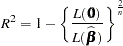where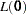is the likelihood of the intercept-only model,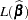is the likelihood of the specified model, andis the sample size. The quantity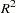achieves a maximum of less than one for discrete models, where the maximum is given by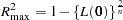To take the frequency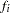and weight
of observationinto account, the sample sizeis replaced inand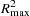with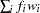. Specifying the NORMALIZE option in the WEIGHT statement makes these coefficients invariant to the scale of the weights.

Nagelkerke (1991) proposes the following adjusted coefficient, which can achieve a maximum value of one: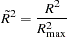Like the AIC and SC statistics described in the section Model Fitting Information,and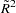are most useful for comparing competing models that are not necessarily nested—larger values indicate better models. More properties and interpretation ofandare provided in Nagelkerke (1991). In the "Testing Global Null Hypothesis: BETA=0" table,is labeled as "RSquare" andis labeled as "Max-rescaled RSquare."   Use the RSQUARE option to requestand.Previous Page | Next Page | Top of Page